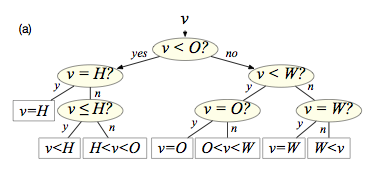## neal young / Chrobak15Optimal

•In 1971, Knuth gave an $$O(n^2)$$-time algorithm for the classic problem of finding an optimal binary search tree. Knuth's algorithm works only for search trees based on 3-way comparisons, while most modern computers support only 2-way comparisons (e.g., $$<$$, $$\le$$, $$=$$, $$\ge$$, and $$>$$). Until this paper, the problem of finding an optimal search tree using 2-way comparisons remained open --- poly-time algorithms were known only for restricted variants. We solve the general case, giving (i) an $$O(n^4)$$-time algorithm and (ii) an $$O(n\log n)$$-time additive-3 approximation algorithm.

For finding optimal binary split trees, we (iii) obtain a linear speedup and (iv) prove some previous work incorrect.
Conference version of [Chrobak22Simple, Chrobak21Cost, Chrobak22Huang]